### 1. Generating observations from a mixture of 3 two-dimensional Gaussians

• we specify the means, standard deviations, correlation coefficients, and weights for all 3 clusters:
mu1 = c(5, 5)   # x and y component of mean value vector for cluster 1
mu2 = c(9, 9)   # x and y component of mean value vector for cluster 2
mu3 = c(8, 5)   # x and y component of mean value vector for cluster 3

sigma1 = c(1.0, 1.1)    # x and y component of standard deviation vector for cluster 1
sigma2 = c(1.0, 1.0)    # x and y component of standard deviation vector for cluster 1
sigma3 = c(1.0, 1.1)    # x and y component of standard deviation vector for cluster 1

rhoxy1 = 0.8    # correlation between x and y, cluster 1
rhoxy2 = 0.9    # correlation between x and y, cluster 2
rhoxy3 = 0.6    # correlation between x and y, cluster 3

alpha = c(0.3, 0.3, 0.4)    # cluster weights
sum(alpha) == 1             # must be TRUE
##  TRUE
• the covariance matrix for cluster k is then constructed using the corresponding standard deviations and correlation coefficients:

$\pmb{\Sigma_k} = \begin{pmatrix} \sigma_x^2 & \rho \sigma_x \sigma_y \\ \rho \sigma_x \sigma_y & \sigma_y^2 \end{pmatrix}$

• (the cluster index k was suppressed in the matrix for better visibility)
• the corresponding R code is:
mat = c(sigma1^2, rhoxy1*sigma1*sigma1, rhoxy1*sigma1*sigma1, sigma1^2)
Sigma1 = matrix(mat, nrow = 2, byrow = TRUE)    # Covariance matrix for cluster 1
mat = c(sigma2^2, rhoxy2*sigma2*sigma2, rhoxy2*sigma2*sigma2, sigma2^2)
Sigma2 = matrix(mat, nrow = 2, byrow = TRUE)    # Covariance matrix for cluster 2
mat = c(sigma3^2, rhoxy3*sigma3*sigma3, rhoxy3*sigma3*sigma3, sigma3^2)
Sigma3 = matrix(mat, nrow = 2, byrow = TRUE)    # Covariance matrix for cluster 3
• the $$\Sigma_k$$ are symmetric, positive (semi-) definite matrices
• once mean vector $$\mu_k$$ and covariance matrix $$\Sigma_k$$ are specified, a sample point from a multivariate Gaussian can be generated by combining samples of standard-normal variables:
• to construct a d-dimensional Gaussian, we have to determine a (d x d) - matrix $$A$$ so that $$A \cdot A^T = \Sigma$$
• the matrix $$A$$ can be calculated from $$\Sigma$$ using Cholesky decomposition
• then, a d-dimensional vector $$z$$ of $$N(0,1)$$ variables is generated using the built-in R-function rnorm()
• finally, the sample point $$w$$ of the multivariate Gaussian is calculated as $$w = A \cdot z + \mu$$
• the function rmultinorm() combines these steps:
rmultinorm <- function(mu, Sigma){
d = length(mu)       # d = dimension
A = t(chol(Sigma))   # determine a matrix A so that A %*% t(A) = Sigma, "Cholesky decomposition"
Z = rnorm(d)         # generate N(0,1) sample point with dimension d
w = A %*% Z + mu     # sample point of the d-dimensional Gaussian
return(w[,1])        # returns sample point of a multivariate Gaussian with mean mu and covariance matrix Sigma
} 
mu = list()
mu[] = mu1
mu[] = mu2
mu[] = mu3

Sigma = list()
Sigma[] = Sigma1
Sigma[] = Sigma2
Sigma[] = Sigma3
• using the rmultinorm() function, we conduct two-step experiment to generate sample points from a 2-D Gaussian mixture (with 3 clusters)
• first we randomly choose one of the 3 clusters
• subsequently, we draw a sample point from the chosen cluster
• this is realized in the following loop:
• (note that all code snippets are designed for comprehensibility, not for efficiency!)
N = 1000   # number of observations to generate
x = matrix(NA, ncol = 2, nrow = N)     # matrix for 1000 2-dim observations, one row = one observation
cols = rep(NA, N)                      # color recalls which Gaussian was chosen
color_palette = c("red", "darkgreen", "blue")   # different color for each cluster

for (i in 1:N) {
component = sample(c(1,2,3), size = 1, prob = alpha)      # draw a cluster with probability alpha
x[i,] = rmultinorm(mu[[component]], Sigma[[component]])   # generate a 2-dim sample from the chosen Gaussian
cols[i] = color_palette[component]                        # assign a color to the sample point
}
dim(x)  # the random sample points are stored in the array x
##  1000    2
• plot the generated sample points
• color indicates the cluster a sample point originated from
• Important: in practice, we do not know from which cluster a sample point originated from (the origins are “hidden variables”)
plot(x[,1], x[,2], col = cols, main = "Observations from Gaussian mixture", font.main = 1)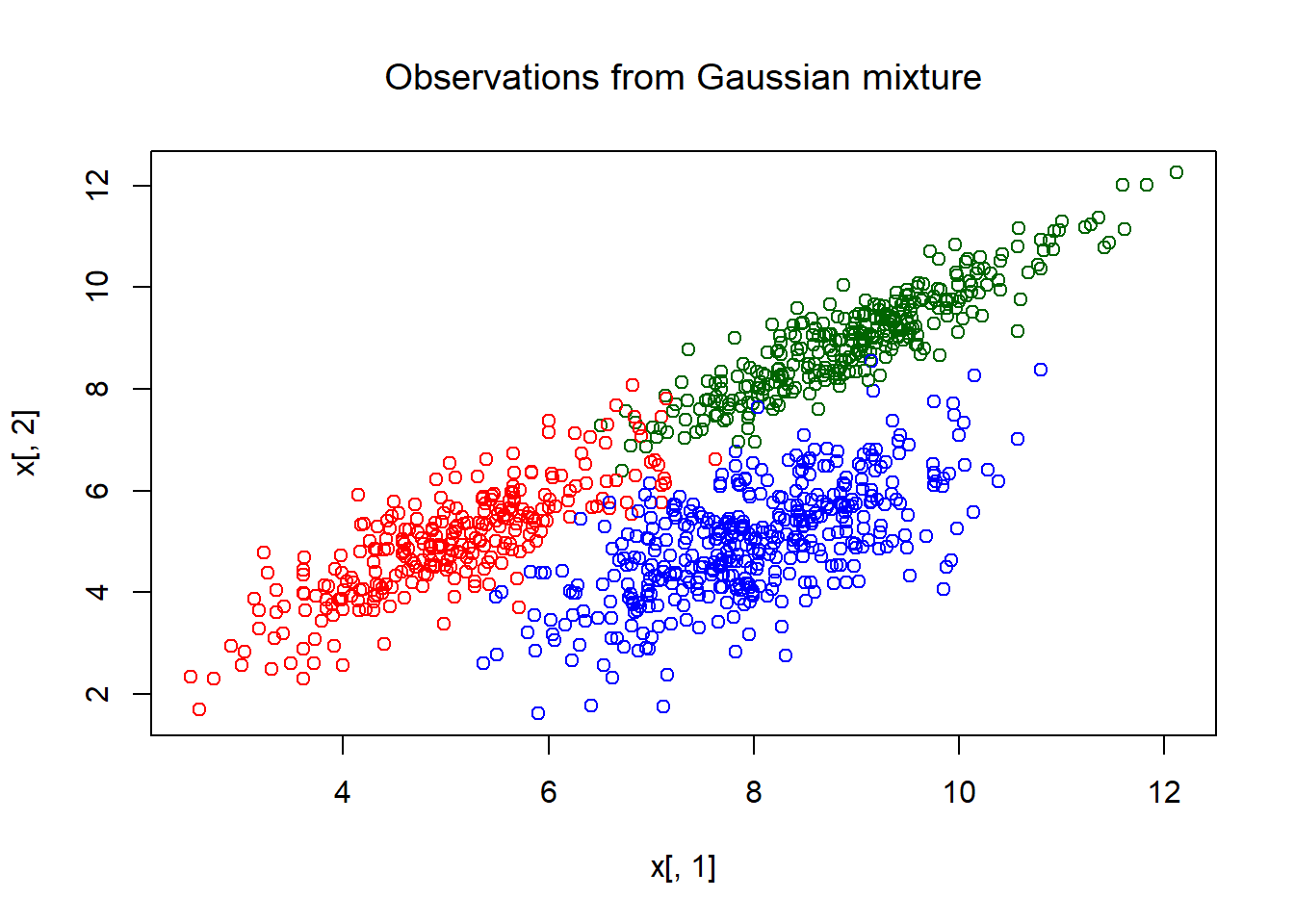### 2. Estimation of the parameters from the sample points using EM

#### 2.1. Initialization

• in practice, the origins of the sample points are not known
• that means we have to guess the parameters from a plot of the sample points without knowledge of the hidden variables (without “color” as above):
plot(x[,1], x[,2], col = "grey", main = "Observations from Gaussian mixture", font.main = 1)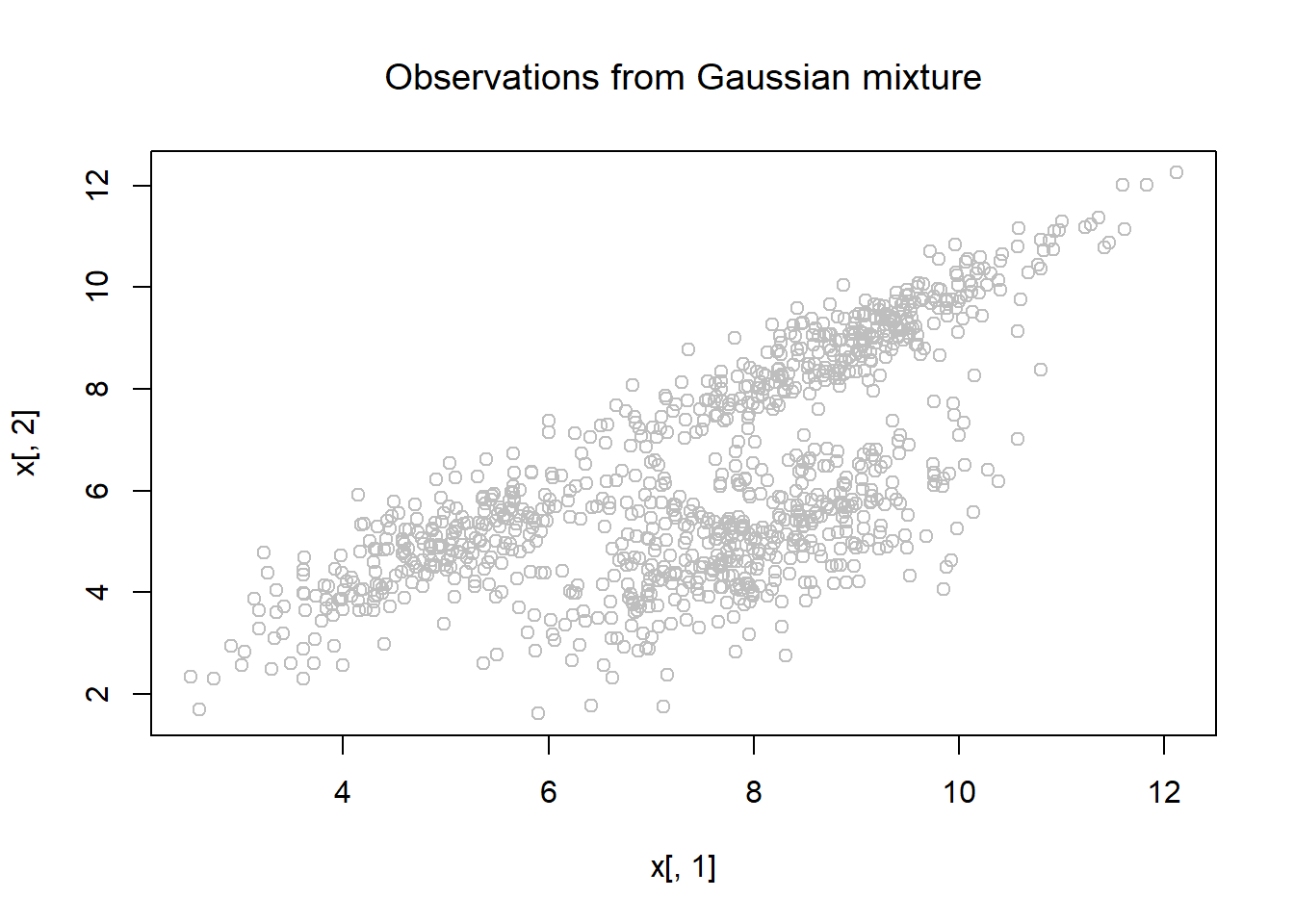• we have to know (or to guess) the number of clusters K the points are produced from
• also, we define how many iterations we want to carry out at most
K = 3           # we have to know (or to guess) the number of Gaussians
max_iter = 50   # maximum number of iterations
• we label the first guess with the additional characters “_t"
• of course, the first guess will only roughly agree with the “true” parameters (here, the true parameters are the ones we used above for sample generation)
mu1_t = c(6, 6)     # x and y component of mean value vector for cluster 1
mu2_t = c(8, 8)     # x and y component of mean value vector for cluster 2
mu3_t = c(7, 6)     # x and y component of mean value vector for cluster 3

sigma1_t = c(1.2, 1.0)    # x and y component of standard deviation vector for cluster 1
sigma2_t = c(1.2, 1.2)    # x and y component of standard deviation vector for cluster 1
sigma3_t = c(1.4, 1.5)    # x and y component of standard deviation vector for cluster 1

rhoxy1_t = 0.7    # correlation between x and y, cluster 1
rhoxy2_t = 0.8    # correlation between x and y, cluster 2
rhoxy3_t = 0.7    # correlation between x and y, cluster 3

alpha_t = c(0.3, 0.3, 0.4)    # cluster weights
sum(alpha_t) == 1             # must be TRUE
##  TRUE
• with that, we have to construct the first guess of the covariance matrices $$\Sigma_k$$:
mat = c(sigma1_t^2, rhoxy1_t*sigma1_t*sigma1_t, rhoxy1_t*sigma1_t*sigma1_t, sigma1_t^2)
Sigma1_t = matrix(mat, nrow = 2, byrow = TRUE)    # Covariance matrix for cluster 1
mat = c(sigma2_t^2, rhoxy2_t*sigma2_t*sigma2_t, rhoxy2_t*sigma2_t*sigma2_t, sigma2_t^2)
Sigma2_t = matrix(mat, nrow = 2, byrow = TRUE)    # Covariance matrix for cluster 2
mat = c(sigma3_t^2, rhoxy3_t*sigma3_t*sigma3_t, rhoxy3_t*sigma3_t*sigma3_t, sigma3_t^2)
Sigma3_t = matrix(mat, nrow = 2, byrow = TRUE)    # Covariance matrix for cluster 3
• collect the mean vectors and covariance matrices in lists:
mu_t = list()
mu_t[] = mu1_t
mu_t[] = mu2_t
mu_t[] = mu3_t

Sigma_t = list()
Sigma_t[] = Sigma1_t
Sigma_t[] = Sigma2_t
Sigma_t[] = Sigma3_t
• it can be interesting to see a contour plot of the probability distribution that follows from our first guess together with the “observed” sample points
• this might give us a clue if the guess if fairly well … or completely aside
• we have to define the grid for the contour plot first:
xmin = 0 ; xmax = 12   # define the plot range by looking at the sample point plot
ymin = 0 ; ymax = 12
x1 = seq(xmin, xmax, length = 200)
x2 = seq(ymin, ymax, length = 200)
• we need also a function that gives us a sample point from a mixture of Gaussian distributions
• the function fx_Gauss() can do that:
fx_Gauss <- function(x, y, mu, Sigma, alpha) {   # Density function for a mixture of 2-D Gaussians
if(length(x) != length(y)) stop("\n 'x' and 'y' must have equal length \n\n")
N = nrow(x)
if(!is.list(mu)) stop("\n 'mu' must be a list with K components.\n\n")
if(!is.list(Sigma)) stop("\n 'Sigma' must be a list with K components.\n\n")
K = length(mu)   # number of clusters
density = 0
for (k in 1:K) density = density + alpha[k]*dmvnorm(cbind(x, y), mean = mu[[k]], sigma = Sigma[[k]])
return(density)
}
• fx_Gauss() uses the function dmvnorm() from the R-package mvtnorm, load the library:
library(mvtnorm)
• using outer, we define a 2-dimensional grid for the contour plot
• we use fx_Gauss() to determine the function value for each grid point
• the function values are based on the first guess (variables mu_t, Sigma_t, alpha_t):
fx_t = outer(x1, x2, FUN = function(x, y) fx_Gauss(x, y, mu = mu_t, Sigma = Sigma_t, alpha = alpha_t))
plot(x[,1], x[,2], col = "grey", main = "Observations from Gaussian mixture", font.main = 1)  # sample points
contour(x1, x2, fx_t, col = "red", lty = 2, add = TRUE)   # contour-plot representing the 1st guess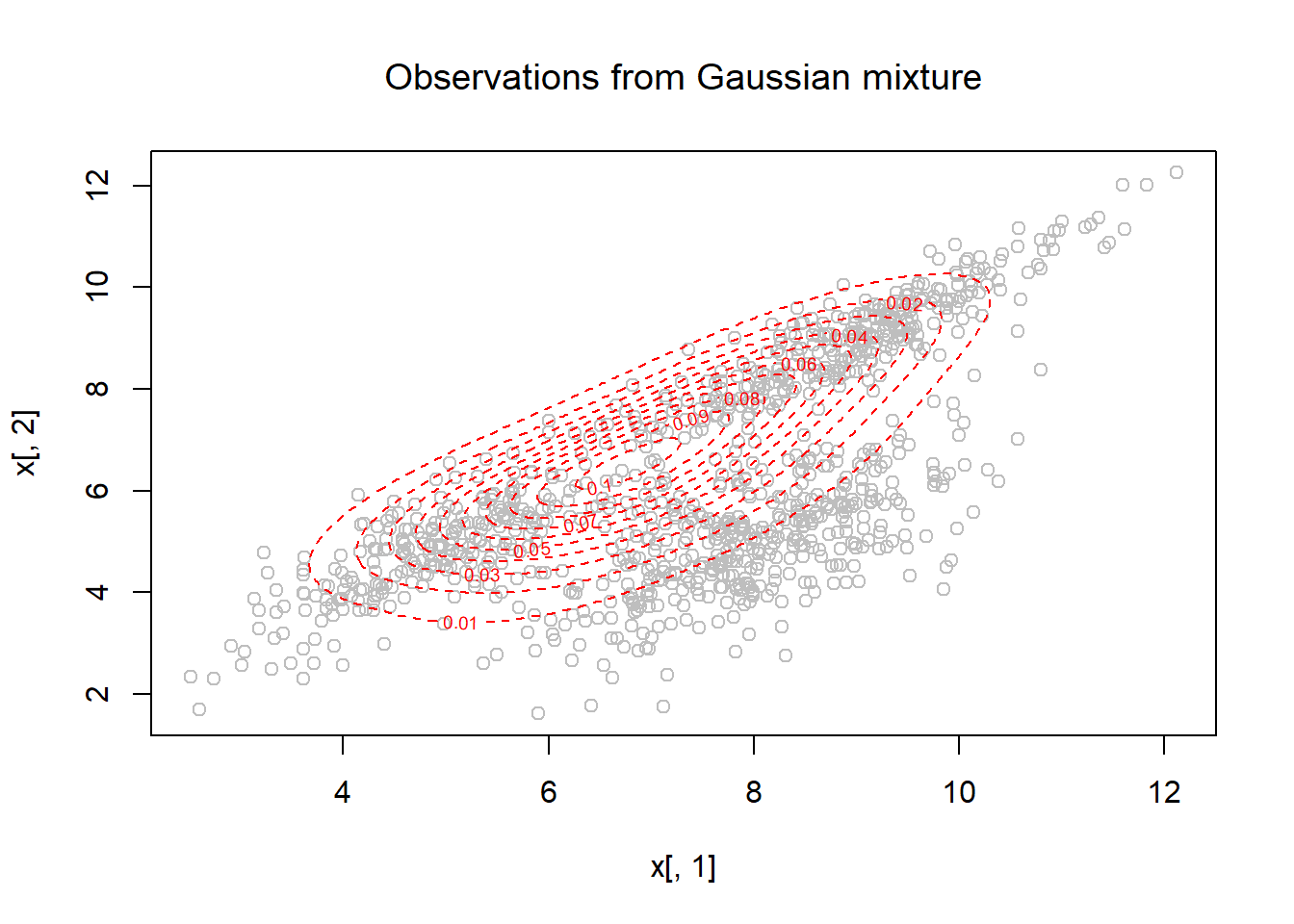• we see that the first guess is not perfect but might work
• because we produced the sample points ourselves, and thereby know the true parameters, we can cheat a little bit and add the contour plots based on these true parameter values:
plot(x[,1], x[,2], col = "grey", main = "Observations from Gaussian mixture", font.main = 1)  # sample points
contour(x1, x2, fx_t, col = "red", lty = 2, add = TRUE)         # contour-plot representing the 1st guess
fx_sol = outer(x1, x2, FUN = function(x, y) fx_Gauss(x, y, mu = mu, Sigma = Sigma, alpha = alpha))
contour(x1, x2, fx_sol, col = "green", lty = 2, add = TRUE)     # contour-plot representing the true prob. distribution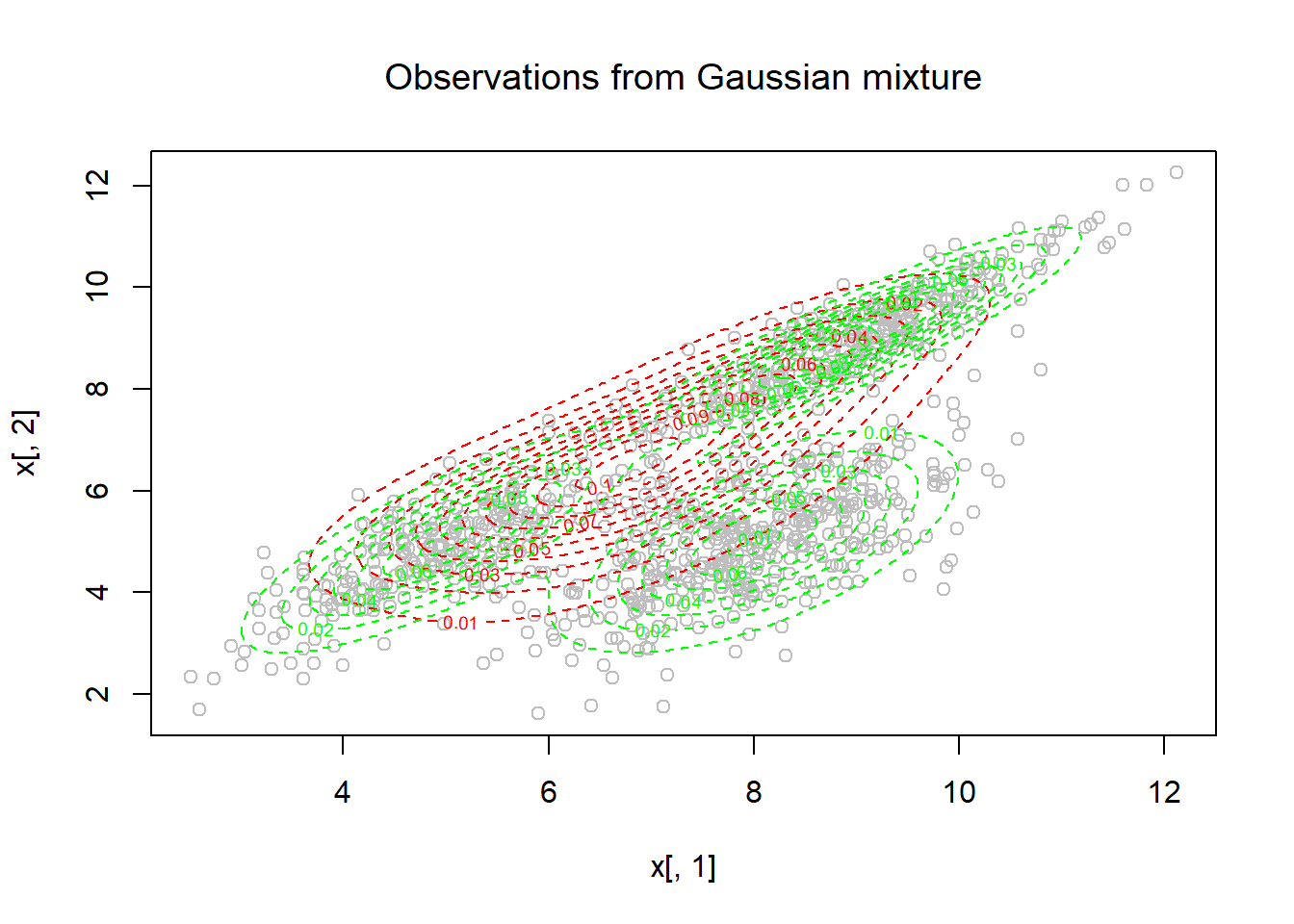• red contour lines stand for the first guess, green contour lines show the true distribution
• the 1st guess is not brilliant but we’ll give it a chance!
• we can calculate the log likelihood of the samples based on the 1st guess:
logL = 0
for (k in 1:K) logL = logL + alpha_t[k] * dmvnorm(x, mu_t[[k]], Sigma_t[[k]])   # k-sum
logL = log(logL)
logL = sum(logL)    # i-sum
cat(paste(" Log likelihood after 1st guess:", signif(logL,4), "\n"))         
##  Log likelihood after 1st guess: -4255
Lvector = logL      # store the first value, append logL-values during the EM loop below
• define data structures for the updated parameters:
mu = list()
mu[] = rep(NA, 2)  # components x and y
mu[] = rep(NA, 2)
mu[] = rep(NA, 2)
Sigma = list()
Sigma[] = matrix(NA, nrow = 2, ncol = 2)
Sigma[] = matrix(NA, nrow = 2, ncol = 2)
Sigma[] = matrix(NA, nrow = 2, ncol = 2)
alpha = rep(NA, K)          # K = number of clusters
• we must not forget to define maximum relative errors for the parameters:
e_mu_max = 0.001       # means
e_Sigma_max = 0.001    # covariance matrices
e_alpha_max = 0.001    # mixture weights

#### 2.2. Looping through E- and M-steps

• now we can alternate through E- and M-step
• (note that all code snippets are designed for comprehensibility, not for efficiency!)
• during the E-step, we calculate the “membership probabilities” for the sample points, $$\omega_{ik}$$:

$\omega_{ik} = \; \frac{ \alpha_k^t \cdot f_k \left( \pmb{x_i} \mid \pmb{\mu_k^t}, \pmb{\Sigma_k^t} \right) }{\sum_{k} \alpha_k^t \cdot f_k \left( \pmb{x_i} \mid \pmb{\mu_k^t}, \pmb{\Sigma_k^t} \right)}$

• during the M-step, we update the parameter values (to maximize $$Q_1(\theta, \theta_t$$):

$\pmb{\mu_m} = \frac{\sum_{i=1}^N \omega_{im} \cdot \pmb{x_i}}{\sum_{i=1}^N \omega_{im}}$

$\pmb{\Sigma_m} = \frac{ \sum_{i=1}^N \omega_{im} \cdot \left(\pmb{x_i} - \pmb{\mu_m} \right) \cdot \left(\pmb{x_i} - \pmb{\mu_m} \right)^T }{ \sum_{i=1}^N \omega_{im}}$

$\alpha_m = \frac{1}{N} \cdot \sum_{i=1}^N \omega_{im}$

for (iter in 1:max_iter) {

# --- E-step
omega = matrix(NA, nrow = N, ncol = K)
for (k in 1:K) omega[,k] = alpha_t[k] * dmvnorm(x, mu_t[[k]], Sigma_t[[k]])       # numerator for each k
denominator  = 0; for (k in 1:K) denominator = denominator + omega[,k]            # denominator
for (k in 1:K) omega[,k] = omega[,k] / denominator
if(!all(round(rowSums(omega),4) == 1)) stop("\n  All rowsums of omega must be 1! \n\n")
if(round(sum(rowSums(omega)), 3) != N)
stop("\n\n  Sum over all elements of omega must be equal to the number of observations! \n\n")
if(any(omega < 0)) stop("\n  No element of omega can be negative! \n\n")

# --- M-step

# update means:
for (k in 1:K) mu[[k]] = as.vector((omega[,k] %*% x) / sum(omega[,k]))   # a vector with 2 components

# update covariance matrices:
for (k in 1:K) {
Sigma[[k]] = matrix(rep(0,4), nrow = 2)     # init
for (i in 1:N) Sigma[[k]] = Sigma[[k]] + omega[i,k] * ((x[i,] - mu_t[[k]]) %*% t(x[i,] - mu_t[[k]]))
Sigma[[k]] = Sigma[[k]] / sum(omega[,k])    # weigthed covariance matrix
}

# update cluster membership
for (k in 1:K) alpha[k] = sum(omega[,k]) / N

# new value for log-likelihood:
logL = rep(0 , N)
for (k in 1:K) logL = logL + alpha[k] * dmvnorm(x, mu[[k]], Sigma[[k]])  # k-sum
logL = log(logL)
logL = sum(logL) # i-sum
cat(paste("Log likelihood after iteration", iter, ":", signif(logL,4), "\n"))
Lvector = c(Lvector, logL)    # append the new value

# calculate relative errors:
e_mu = max(abs((unlist(mu) - unlist(mu_t))/(unlist(mu) + 1e-50)))
e_Sigma = max(abs((unlist(Sigma) - unlist(Sigma_t))/(unlist(Sigma) + 1e-50)))
e_alpha  = max(abs((alpha - alpha_t) / (alpha_t + 1e-50)))
cat(paste("Loop", iter, ":  e_mu =", signif(e_mu,4), "e_Sigma =", signif(e_Sigma,4), "e_alpha =", signif(e_alpha,5), "\n"))

# need another loop?:
cond1 = e_mu <= e_mu_max          # condition 1, for mu
cond2 = e_Sigma <= e_Sigma_max    # condition 2, for sigma
cond3 = e_alpha <= e_alpha_max    # condition 3, for alpha
if(cond1 & cond2 & cond3) break   # converged!

# otherwise, continue to next loop:
mu_t = mu
Sigma_t = Sigma
alpha_t = alpha

} # EM-loop
## Log likelihood after iteration 1 : -3633
## Loop 1 :  e_mu = 0.1521 e_Sigma = 0.4903 e_alpha = 0.375
## Log likelihood after iteration 2 : -3509
## Loop 2 :  e_mu = 0.06336 e_Sigma = 0.6493 e_alpha = 0.058626
## Log likelihood after iteration 3 : -3461
## Loop 3 :  e_mu = 0.0401 e_Sigma = 0.4534 e_alpha = 0.081443
## Log likelihood after iteration 4 : -3438
## Loop 4 :  e_mu = 0.01227 e_Sigma = 0.2887 e_alpha = 0.074074
## Log likelihood after iteration 5 : -3427
## Loop 5 :  e_mu = 0.009132 e_Sigma = 0.1364 e_alpha = 0.053024
## Log likelihood after iteration 6 : -3420
## Loop 6 :  e_mu = 0.006481 e_Sigma = 0.08775 e_alpha = 0.038344
## Log likelihood after iteration 7 : -3416
## Loop 7 :  e_mu = 0.004695 e_Sigma = 0.05418 e_alpha = 0.028112
## Log likelihood after iteration 8 : -3414
## Loop 8 :  e_mu = 0.004269 e_Sigma = 0.03442 e_alpha = 0.020268
## Log likelihood after iteration 9 : -3413
## Loop 9 :  e_mu = 0.003457 e_Sigma = 0.02189 e_alpha = 0.014391
## Log likelihood after iteration 10 : -3412
## Loop 10 :  e_mu = 0.002669 e_Sigma = 0.01588 e_alpha = 0.010161
## Log likelihood after iteration 11 : -3412
## Loop 11 :  e_mu = 0.002013 e_Sigma = 0.01293 e_alpha = 0.007187
## Log likelihood after iteration 12 : -3412
## Loop 12 :  e_mu = 0.001502 e_Sigma = 0.01015 e_alpha = 0.0051119
## Log likelihood after iteration 13 : -3412
## Loop 13 :  e_mu = 0.001114 e_Sigma = 0.007804 e_alpha = 0.0036597
## Log likelihood after iteration 14 : -3412
## Loop 14 :  e_mu = 0.0008249 e_Sigma = 0.00593 e_alpha = 0.0026365
## Log likelihood after iteration 15 : -3412
## Loop 15 :  e_mu = 0.0006105 e_Sigma = 0.004475 e_alpha = 0.00191
## Log likelihood after iteration 16 : -3412
## Loop 16 :  e_mu = 0.0004521 e_Sigma = 0.003365 e_alpha = 0.0013903
## Log likelihood after iteration 17 : -3412
## Loop 17 :  e_mu = 0.0003352 e_Sigma = 0.002525 e_alpha = 0.0010161
## Log likelihood after iteration 18 : -3412
## Loop 18 :  e_mu = 0.0002488 e_Sigma = 0.001893 e_alpha = 0.00074533
## Log likelihood after iteration 19 : -3412
## Loop 19 :  e_mu = 0.0001849 e_Sigma = 0.001419 e_alpha = 0.00054837
## Log likelihood after iteration 20 : -3412
## Loop 20 :  e_mu = 0.0001376 e_Sigma = 0.001063 e_alpha = 0.00040454
## Log likelihood after iteration 21 : -3412
## Loop 21 :  e_mu = 0.0001025 e_Sigma = 0.0007965 e_alpha = 0.00029913

### 3. Looking at the results

• output the calculated parameter values (which should be fairly close to the true parameters)
• scatterplot of the log-likelihood vs. iteration (likelihood cannot decrease according to the theory)
• a plot of the sample points together with the obtained solution
• a heat plot of the obtained solution
• a 3D-plot of the obtained solution
if(iter == max_iter) {
warning("Not converged!\n\n")
} else {
cat("Converged after", iter, "iterations.\n\n")
}
## Converged after 21 iterations.
## List results:
cat(paste("Mean values of the", K, "clusters:\n")) 
## Mean values of the 3 clusters:
for (k in 1:K) {
mux = signif(mu[[k]],4)
muy = signif(mu[[k]],4)
cat(paste("  Cluster", k, "  mux =", mux, "  muy =", muy, "\n"))
}
##   Cluster 1   mux = 4.975   muy = 4.933
##   Cluster 2   mux = 8.947   muy = 8.937
##   Cluster 3   mux = 7.967   muy = 5.002
cat(paste("Standard deviations and correlation of the", K, "clusters:\n"))
## Standard deviations and correlation of the 3 clusters:
for (k in 1:K) {
sigmax = signif(sqrt(Sigma[[k]][1,1]),4)
sigmay = signif(sqrt(Sigma[[k]][2,2]),4)
rhoxy  = signif(Sigma[[k]][1,2]/sigmax/sigmay, 4)
cat(paste("  Cluster", k, "  sdx =", sigmax, "  sdy =", sigmay, "  rhoxy =", rhoxy, "\n"))
}
##   Cluster 1   sdx = 0.9608   sdy = 1.067   rhoxy = 0.8153
##   Cluster 2   sdx = 1.056   sdy = 1.053   rhoxy = 0.9087
##   Cluster 3   sdx = 1.018   sdy = 1.117   rhoxy = 0.6334
cat(paste("Cluster weigths of the", K, "clusters:\n"))
## Cluster weigths of the 3 clusters:
for (k in 1:K) cat(paste("  Cluster", k, "  alpha =", signif(alpha[k], 4), "\n"))
##   Cluster 1   alpha = 0.2658
##   Cluster 2   alpha = 0.3149
##   Cluster 3   alpha = 0.4193
• a plot of the log-likelihood vs. iteration number
• according to the theory, the log-likelihood can never decrease during iterations
  plot(Lvector, ylab = "log(L)", main = "Log-likelihood", font.main = 1, xlab = "iter", col = "blue", lwd = 1.5 )
mtext(side = 3, "First value based on first guess", col = "blue", cex = 0.9)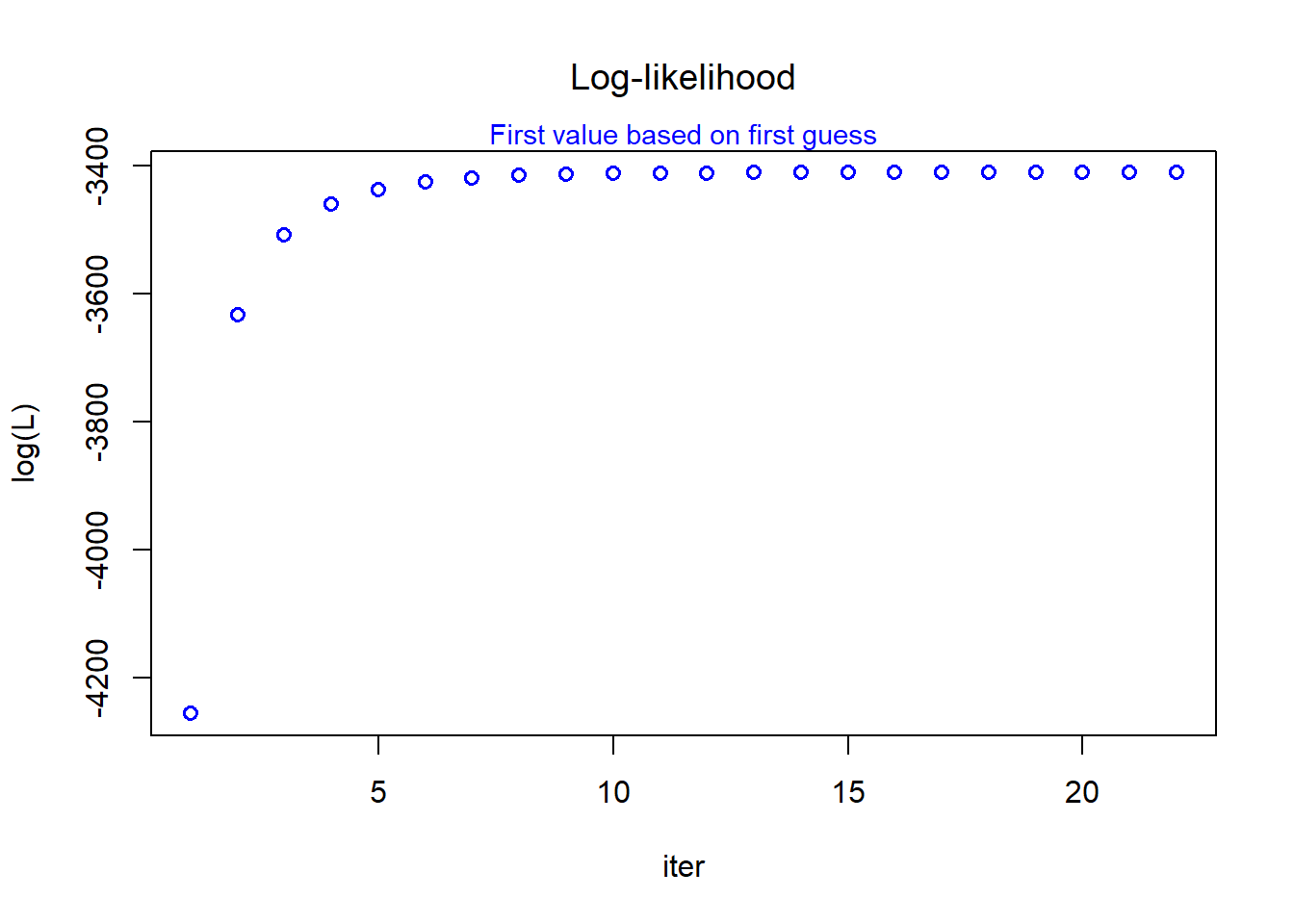• a plot of the sample points plus contour lines representing the solution that has been found:
  plot(x[,1], x[,2], xlab = "x1", ylab = "x2", col = "grey", main = "Sample points and EM solution", font.main = 1)

# define grid for the contour plot (same as above):
xmin = 0 ; xmax = 12
ymin = 0 ; ymax = 12
x1 = seq(xmin, xmax, length = 200)
x2 = seq(ymin, ymax, length = 200)
fx_sol = outer(x1, x2, FUN = function(x, y) fx_Gauss(x, y, mu = mu, Sigma = Sigma, alpha = alpha))
contour(x1, x2, fx_sol, col = "green", lty = 2, add = TRUE)   # contour-plot showing the calculated solution`